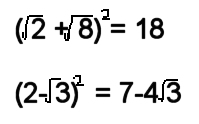# Maths. Surds. Help.

Two example problems.The squiggly lines are square root. The power is 2 in both cases.

Is it possible to explain those two ‘sums’ ? I am finding them incomprehensible.

## 18 thoughts on “Maths. Surds. Help.”

1.dino says:

I haven’t tried this out yet but here goes:

1. Find the square root of 2 and 8. Add the answers together and multiply this second answer by itself. This should equal 18.

2.(Part 1) Find the square root of 3. Take the answer away from 2 and multiply this second answer by itself. I’ll call that A.
(Part 2) Find the square root of 3. Multiply that by 4 and subtract the answer from 7. I’ll call that b.
If the equation is correct then A should be equal to B.

I’ll go try them and see if they work now 🙂

2.SteveAgl says:

I suppose it was a test that asking you to insert some math symbols will make the sums correct, right ?
If you try to calculate them with a calculator you will see that the square root of 2 plus the square root of 8, all at the power of 2 give you 18. I suppose the other it’s a the same.

Maybe i’m missing what is your real question.

3.Mark says:

Sorry – I should have said: This is part of a NON-calculator maths test.

4.dino says:

Yep it works. How close depends on how many decimal places you work to:

1.
(1.4142+2.8284)x(1.4142+2.8284)=18

2.
(2-1.7321)x(2-1.7321)=0.072 (A)
7-(4×1.7321)=0.072 (B)

A=B

🙂

5.dino says:

“This is part of a NON-calculator maths test. ”

JESUS!!!!

The actual calc is fine except how the hell do you work out a square root without a calculator. I guess back in the dim and distant past they could do it but I haven’t a clue!

6.David says:

(a+b)^2 = a^2 + b^2 + 2ab

(SQR(2)+sqr(8))^2 = 2+8+2*(sqr(2)*sqr(8)), but sqr(8) = 2*sqr(2)
so 2+8+2*(sqr(2)*sqr(8)) = 2+8+2(sqr(2)*2*sqr(2)) = 10+2*(4)
= 18

(a-b)^2 = a^2+b^2-2ab
=> (2-sqr(3))^2 = 4+3-2*2*sqr(3) = 7-4*sqr(3)

7.Mark says:

David – that is a WOW from me! I will of course be telling D that maths like this is invaluable in many aspects of day-to-day life and as such she should listen carefully to her teachers 🙂

8.Jennifer says:
9.Jennifer says:
10.fluffy says:

When you start trying to work out square roots of negative numbers then things start getting interesting 🙂

11.Cameron aka desk003 says:

You make my brain hurt.

12.jammer says:

David on 13:28 Thursday 8 Dec 2005 at 13:28 Thursday 8 Dec said:

(a+b)^2 = a^2 + b^2 + 2ab

(SQR(2)+sqr(8))^2 = 2+8+2*(sqr(2)*sqr(8)), but sqr(8) = 2*sqr(2)
so 2+8+2*(sqr(2)*sqr(8)) = 2+8+2(sqr(2)*2*sqr(2)) = 10+2*(4)
= 18

(a-b)^2 = a^2+b^2-2ab
=> (2-sqr(3))^2 = 4+3-2*2*sqr(3) = 7-4*sqr(3)

Sorry for poor english, i’m from Russia
bravo:)) i was afraid that nobody will remebered this school rule. It’s the elementary case of using Isaak Newton(right or no?) binom

13.gruppo says:

Guter Aufstellungsort, ja!

14.gua[p says:

putang ina ng mga tao dto